This is the discussion forum for OxyPlot.
For bugs and new features, use the issue tracker located at GitHub.
Also try the chat room!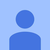+3
На рассмотрении

###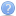Is it possible to draw spectrogram with OxyPlot ?

Daniel Daniel 5 лет назад • обновлен Oystein Bjorke 5 лет назад
Drawing spectrogram.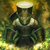+3
На рассмотрении

###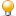Thread safety and RenderPoints

Nick Donais 5 лет назад • обновлен Joshua 3 месяца назад
So I've seen the error a few times, mainly when the plot is trying to update and data is being added to the series:

> System.InvalidOperationException occurred
> HResult=-2146233079
> Message=Collection was modified; enumeration operation may not execute.
> Source=mscorlib
> StackTrace:
> at System.Collections.Generic.List`1.Enumerator.MoveNextRare()

Seems to be because ICollection is being used here:
https://github.com/oxyplot/oxyplot/blob/develop/So...
Would it be better to use one of the concurrent collects for this?+2

###Heatmap with non equidistant values

Rainer Worbis 5 лет назад • обновлен Augustin Šulc 2 года назад
Is there a possibility to draw a heatmap where the provided values are not spaced equally but in arbitary distances?

Thanks,
Rainer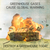+2

###Rotate series labels.

bkboggy 4 года назад
Does anyone know how I can rotate labels? I need it in my situation, where a graph may have a large number of columns, to prevent overlapping of labels, which are at the top of each column. Here's my graph: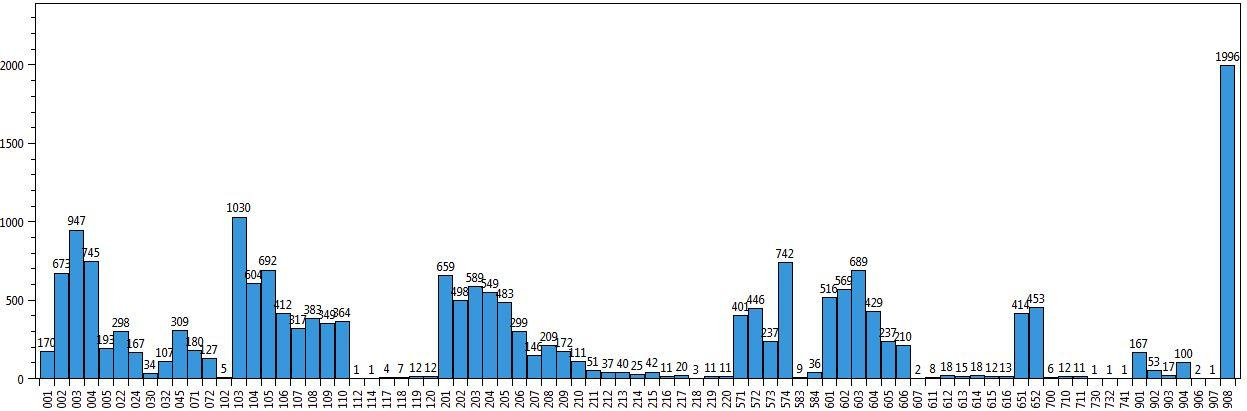That's actually a demonstration of best-case scenario, where there's not a whole lot over spilling going on from labels... but if the graph is shrunk, labels begin to be cut-off. I was able to rate the category axis by setting its angle to -90 degrees, but I can't find any way to do the same thing with labels. I've looked through the source code, documentation and examples.+2
На рассмотрении

###Areaseries: Fill area below baseline (realtime)

Steve 4 года назад • обновлен Oystein Bjorke 4 года назад
Hi,
currently I'm implementing a realtime chart and so far I have the following: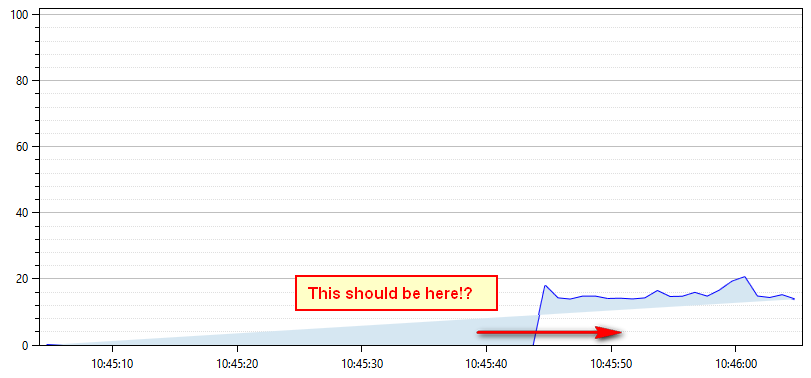But I want something like this: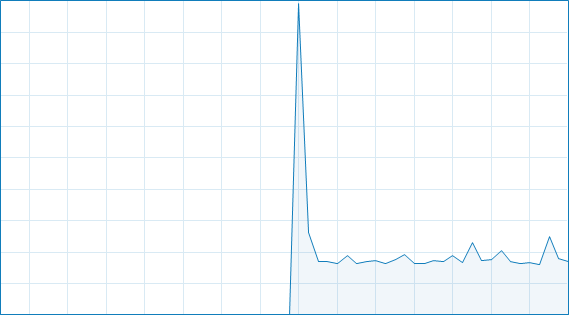Any ideas how to achieve this? Here's the source code:

```/// <summary>
/// Setup Plot
/// </summary>
private void SetupPlot()
{
this.time = DateTime.Now;

this.Plot = new PlotModel();

this.Plot.Axes.Add(new LinearAxis()
{
IsZoomEnabled = false,
Maximum = 102,
Minimum=0,
MajorGridlineStyle = LineStyle.Solid,
MinorGridlineStyle = LineStyle.Dot,
Position = AxisPosition.Left
});

this.Plot.Axes.Add(new DateTimeAxis()
{
IsZoomEnabled = false,
Position = AxisPosition.Bottom,
});

var areaSeries = new AreaSeries()
{
StrokeThickness = 2,
LineStyle = OxyPlot.LineStyle.Solid,
Color = OxyColors.Blue,
Color2 = OxyColors.Transparent,
Fill = OxyColor.FromRgb(214, 231, 242),
DataFieldX2 = "X",
ConstantY2 = 0
};

// Fill series with initial values
for (int i = 0; i < 60; i++)
{
areaSeries.Points.Add(new DataPoint(DateTimeAxis.ToDouble(time.Subtract(new TimeSpan(0, 0, 60 - i))), 0));
}

this.Plot.Series.Add(areaSeries);
}```
Update of the Plot (every second):
```void timer_Tick(object sender, EventArgs e)
{
var areaSeries = (AreaSeries)this.Plot.Series;

if (areaSeries.Points.Count > 60)
{
areaSeries.Points.RemoveAt(0);
}

// Update-Plot
double percentage = GetPercentage();

areaSeries.Points.Add(new DataPoint(DateTimeAxis.ToDouble(this.time), percentage));
time = time.AddSeconds(1);

this.Plot.InvalidatePlot(true);
}```
Many Thanks!+2

###Changing axis scale

Rob Maher 5 лет назад
Hi,

I am trying to use OxyPlot to show some data (*shock*).  My question is - how can the axis scale be changed once the data is plotted into it.

For example.  I use the following to create add the data to the graph

public void addDataToGraph()
{
plotModel1.Title = "Data";
linearAxis1.TickStyle = OxyPlot.Axes.TickStyle.Inside;
linearAxis1.IsPanEnabled = true;
linearAxis1.IsZoomEnabled = true;
plotModel1.Axes.Add(linearAxis1);

linearAxis2.Position = AxisPosition.Bottom;
linearAxis2.TickStyle = OxyPlot.Axes.TickStyle.Inside;
linearAxis2.IsPanEnabled = true;
linearAxis2.IsZoomEnabled = true;

plotModel1.Axes.Add(linearAxis2);

plotModel1.Series.Add(spectrum.OxyPlotDataSeries);
plot1.Model = plotModel1;
}

and I want something like

public void Rescale()
{
linearAxis1.Minimum = 100;
linearAxis1.Maximum = 3200;

linearAxis2.Minimum = 200;
linearAxis2.Maximum = 2000;
plot1.Refresh();
plot1.InvalidatePlot(true);

plot1.Model.InvalidatePlot(true);
}

But this doesn't seem to do anything.  What am I missing here?  Any suggestions?

Thanks for looking.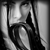+1

###how to invisibility one plotview

future0723 1 год назад

hi, everybody.

Relation to the  Example:"Gridline cropping" ,the plotview was split to four parts,how can I invisibility one of the four?

thanks.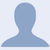+1

###Heatmap Series Realtime

Viraj Patel 3 года назад

I'm looking to create a heatmap series that adds data points in realtime. Does anyone have a working example using the heatmap series?+1
На рассмотрении

###Remove the alpha from 2015.1

Thomas Ibel 4 года назад • обновлен Oystein Bjorke 4 года назад
I think it is time to move the 2015.1 alpha to an official release.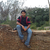+1

###Barseries with reference line on the bar

rakesh murthy 4 года назад
Hello everyone,

I have a cluster Bar series with 2 clusters or may 3 clusters. Now I want one more reference line, like we have on the bullet chart on these clustered bar series. I think its combining the cluster bar series feature and reference line feature of bullet chart.

Please see the picture for the kind of graph I am looking for. Is this possible in oxyplot? if so, please can anybody provide me sample code ?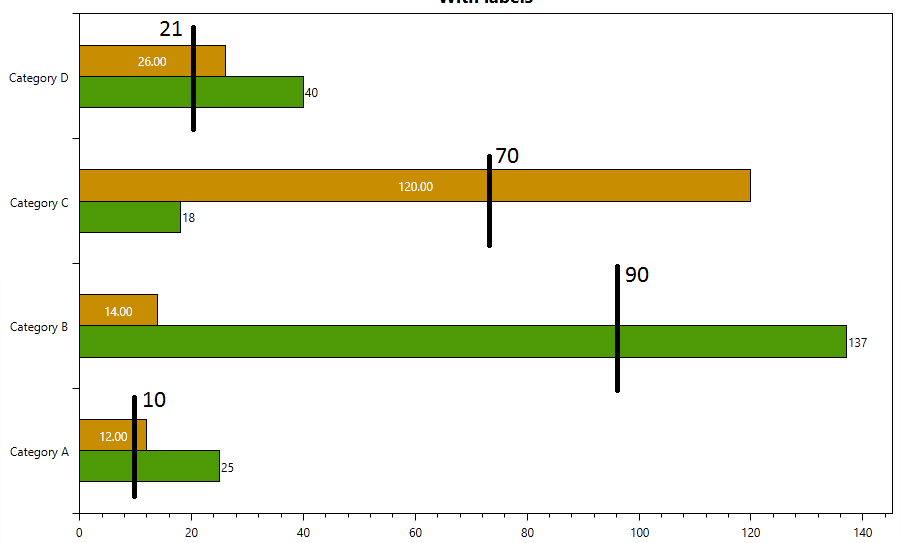﻿

Сервис поддержки клиентов работает на платформе UserEcho